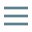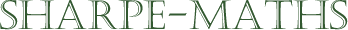# Glossary of terms

## B

•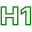### BIDMAS

•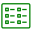BIDMAS is an acrostic, where each letter refers to the operations in the order they should be carried out.

Brackets
Indices
Division
Multiplication
Subtraction

There are many variants of this. The first I learned was BODMAS (the O stood for Order, which means the same as index, power or exponent. However, it is only really used in higher level mathematics, these days, where we talk about the order of a polynomial.)

I had a colleague who used BOMDAS (the M and the D being reversed, makes no difference at all, mathematically.) This also has the advantage of sounding very funny. It generally gets a laugh in class. It is also worth noting that BOMDSA would also work though it is virtually impossible to say which is why nobody uses it.

•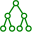•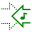•### Brackets

•Brackets are used in mathematics, to make otherwise ambiguous expressions, more obvious in their meaning. In addition, they can be used to change the meaning of an expression.

eg. The following expression correctly gives the answer as $14$, $2 + 3 \times 4 = 14$, because according to BIDMAS, the multiplication must be performed before the addition. However, if we insert brackets around the $2+3$, this changes the value as follows: $(2+3) \times 4 = 20$, as now, the bracket must be evaluated first.

••